﻿ Numerical Investigation of the Turbulence Models Effect on the Combustion Characteristics in a Non-Premixed Turbulent Flame Methane-AirPublications are Open
Access in this journal
Article Versions
Export Article
• Normal Style
• MLA Style
• APA Style
• Chicago Style
Research Article
Open Access Peer-reviewed

### Numerical Investigation of the Turbulence Models Effect on the Combustion Characteristics in a Non-Premixed Turbulent Flame Methane-Air

O. Moussa , Z. Driss
American Journal of Energy Research. 2017, 5(3), 85-93. DOI: 10.12691/ajer-5-3-3
Published online: November 18, 2017

### Abstract

A two-dimensional axis-symmetric numerical model was solved to investigate the effect of four turbulence models on combustion characteristics, such as the velocity, the pressure, the turbulent kinetic energy and the dissipation rate in a methane-air no-premixed flame. Based on the commercial CFD code Ansys fluent 17.0, different turbulence models including the standard k-ε model, the RNG k-ε model, the realizable k-ε model and the standard k-ω model were used to simulate the flow field in a simple burner. The eddy dissipation model with the global reaction schema was applied to model the turbulence reaction interaction in the flame region. A finite volume approach was used to solve the Navier-Stokes equations with the combustion model. Particularly, the effect of these turbulence models on the combustion characteristics was analyzed. The numerical predictions were validated by comparison with anterior experimental results. Moreover, the predicted axial and radial gradients of velocity in the standard k-ε are overall agreement with literature results.

### 1. Introduction

The combustion is a widespread phenomenon for converting chemical energy into heat energy. Although the benefits of combustion (generation of electric power; destruction of waste heat production ...), it emitted toxic gases to the environment, which are thrown into the air by power plants and industrial facilities. On the other side, turbulent no-premixed combustion is widely employed in industrial application. So, the practical combustion systems such as combustion furnaces, rocket engine, gas turbine and diesel engine, use diffusion flame due to its better flame stability, wide operating range, and safety as compared to a premixed flame. In the process, oxidizer and fuel are injected separately into the flame to limit the risk of explosion. Several research showed the field turbulent diffusion flame playing an important role in reducing atmospheric pollution and maintain a more stable flame, therefore a good performance of combustion system. But, there is a large challenge in the experimental studies on complex flow field and turbulence process. Recently, due to the rapid development of the computer technology and advanced numerical algorithm, the technology of computational fluid dynamics (CFD) has been receiving a great progress, which provides reliable tools and conveniences for the researchers of complex fluid movement, such as turbulent flow and combustion. For example, Fu et al. 1 used the four kinds of the two-equation turbulence model such as the standard k-ε, the RNG k-ε, the realizable k-ε and the SST k-ω models to calculate the flow field of the diesel particulate filter with porous media and swirl-type regeneration burner. The numerical results showed the flow characteristics and back-flow features of the Realizable k-ε model are clearer than that of the standard k-ε model and the RNG k-ε model. The maximum peaks of turbulent kinetic energy and the larger gradient are gotten by the RNG k-ε model. The better back-flow characteristics and more abundant details of flow field are found for the SST k-ω model. Ridluan et al. 2 adopted the standard k-ε, the RNG k-ε, the SST k-ω and the RSM turbulence models to get the back-flow characteristics and the distribution of the velocity, the pressure and the turbulent kinetic energy in the three-dimensional turbulent flow of swirl combustor. They confirmed that the standard k-ε turbulence model was poorly effective on the simulation of the annular back-flow region. However, the SST k-ω model and the RNG k-ε model had good prediction performance on swirl flow, and the RSM model has a higher accuracy for this kind of flow. Based on the fluent software, Kucukgokoglan et al. 3 took advantages of the three turbulence models of k-ε (the standard k-ε, the RNG k-ε, and the realizable k-ε) to analyze the performance of the turbulent isothermal flow with a swirl in a furnace at several burners. Then, they compared the results of numerical simulation and experimental data. They noted a great similarity between the numerical results and the experimental data. However, the accuracy of the results is limited to the main characteristics of the flow and depends on the choice of the fine mesh areas near the burner. A numerical study of the effect of tow turbulence models including RNG k-ε model and RSM model on combustion characteristics such as temperature and gas concentration distribution in propane-hydrogen diffusion flame was presented by Yilmaz et al. 4. They validated the numerical method by comparing the velocity profiles, radial flame temperature, and gas concentration distribution with published experimental results. Benim 5 compared the performance of tow turbulence models including the standard k-ε and RNG k-ε turbulence models in a swirling combustor. He found that the RNG k-ε model presents similarity with experiment. However, the standard k-ε model has been compared poorly with the experimental data. Yapici et al. 6 used the RNG k-ε turbulence model to improve the effects of air/fuel ratio and swirl velocity on the combustion of methane-air. Numerical modeling of a turbulent natural gas flow through a non-premixed industrial burner of a slab preheating furnace was studied by Reis et al. 7. They presented a comparison of different turbulence models such as standard k-ε, RNG k-ε, realizable k-ε and Reynolds stress model (RSM) in pressure drop, velocity profiles, and orifice discharge coefficients. The outcomes of the simulation are compared with the experimental results and they found that the Realizable k-ε model appears to be a correct turbulence model. In fact, this model provides results that are relatively similar to the RSM and the RNG k-ε models, requiring only slightly more computational power than the standard k-ε model. Further works such as Raihi et al. 8 selected the standard k-ε model to provide a numerical investigation of the turbulent methane-air jet in a coaxial burner in order to determinate the effect of the air flow on the distribution of the temperature, the gas consumption, and the emission of NOx. The comparison between the numerical prediction of radial temperature gradient and experimental measurement reported by Brookes et al. 9 demonstrated that the standard k-ε turbulent model presents supplementary realistic results of a qualitative and quantitative manner. Benzitouni et al. 10 presented a contribution to the numerical study of the axisymmetric turbulent free jets of non-reactive and reactive fluids, as one can meet them in the burners of the Bunsen types. They noted that the solution of the flow is not reagent, by using the k-ε model which gives results realistic in a qualitative but no quantitative way. Indeed, this model of turbulence is known for the fact that it over-estimates turbulence from where the shift has been noted between experimental results and those obtained in simulation.

According to these anterior researches, it’s clear that the turbulence model has a direct effect on the choice of the numerical model using for the characterization of the combustion characteristics. For thus, this study presents an aerodynamic characterization of a methane-air diffusion flame in a combustion chamber. Particularly, we are interested in the effect of four turbulence models including the standard k-ε, the RNG k-ε, the realizable k-ε and the standard k-ω models.

### 2. Geometric Parameters

Figure 1 presents the physical system consisting of the natural gas (CH4) combustion chamber. The cylindrical combustion chamber has a length L=1000 mm in the axial direction and a radius R=250 mm in the radial direction. The natural gas is introduced into the combustion chamber by an injector aligned with the symmetry line of the chamber, producing a non-premixed flame.

• Figure 1. Combustion chamber used in this study

The burner supplied the necessary quantity of methane as shown in Figure 2, which is defined by a diameter d=6 mm and a length l=200 mm. According to the axis-symmetric problem, we are limited in the present investigation on the 2D computational domain. This geometrical configuration has been adopted by the experimental study of Hraiech et al. 11

• Figure 2. Sketch of the burner

### 3. Numerical Modeling

3.1. Mathematical Formulation

The mathematical modeling of a reactive turbulent flow is carried out using the resolution of the partial differential equations to the nonlinear derivative which expresses the principles of conservation of mass, momentum, chemical species and energy equations for a simple burner in ambient air.

The equation of the mass conservation is written as follows:(1)

Whereis the density and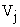presents the component of the instantaneous velocity in the direction j (j = 1, 2, 3).

The equation of the averaged momentum is written as follows: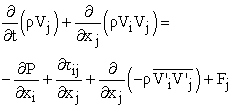(2)

Where the stress tensor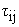and the components of the tensor of Reynolds stresses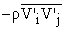are expressed by:(3)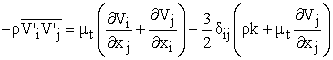(4)

Where p is the pressure,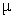is the dynamic viscosity of the fluid and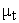is the turbulent viscosity.

The energy equation is written as follows:(5)

Where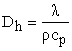is the thermal diffusivity,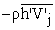is the correlation term between the velocity fluctuation and h is the specific enthalpy, which is defined by the mass enthalpy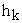for each species presented by: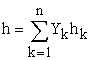(6)

The equation of chemical species conservation for a reactive mixture of n species is shown as follows: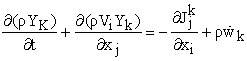(7)

Where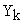,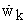,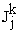are respectively the mass fraction, the production rate of the species k and the mass diffusion flux k in the j direction.

To complete the problem formulation the chemical equation for the combustion of methane in air using a global one-step has been taken by this equation: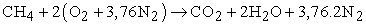(8)
3.2. Turbulence Modeling

In turbulent regime, the introduction of the overall averages of Reynolds revealed new unknown factors coming from the non-linearity of the Navier-Stokes equations, the turbulent constraints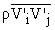and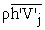corresponding respectively to the term of correlation between the fluctuations speeds in directions i and j and the term of correlation between the fluctuation speed and the mass enthalpy. The closing of the equations system requires the determination of these turbulent constraints, which is the object of the various models of turbulence. In this study, we are interested in the standard k-ε model 12, 13, the RNG k-ε model 14, the Realizable k-ε model 15 and the standard k-ω model 16. These models are widely used in the complex flow field simulation 17, 18.

3.2.1. Standard k-ε Turbulence Model

The standard k-ε turbulence model is composed by two equations. The first is the equation of the turbulent kinetic energy and the second provides the scale length characteristic of the turbulent field. The transport equations for the turbulent kinetic energy k and the dissipation rate of the turbulent kinetic energy ε are written as follows:(9)(10)

Where the turbulent kinetic energy production term and the turbulent viscosity are defined by: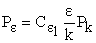(11)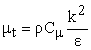(12)

3.2.2. RNG k-ε Turbulence Model

The RNG k-ε turbulence model is based on the method of Renormalisation Group (RNG). This theory gives a model with different constants from those of the standard model, as well as the presence of additional terms in the transport equation of ε. Compared with the standard k-ε model, the RNG k-ε model presents the influence of the turbulent eddies and provides an analytic expression for turbulent Prandtl number. The dissipation rate equation is shown as follows: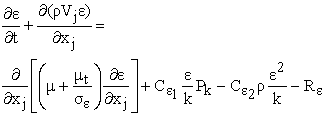(13)

Where: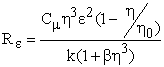(14)

3.2.3. Realizable k-ε Turbulence Model

Compared with the standard k-ε turbulence model, the realizable k-ε model differs in two mains. Firstly, the realizable k-ε model contains a new formulation for the turbulent viscosity. Secondly, a new transport equation for the dissipation rate has been added which is derived from a precise equation for the fluctuation of laminar flows speed 19. The turbulent kinetic energy and dissipation rate transport equations are defined by:(15)(16)

Where: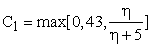(17)(18)

In this model, the viscosity coefficient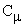is a function of the average strain rate and the curl. It is computed from: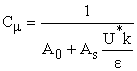(19)

Where:(20)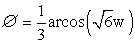(21)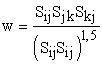(22)(23)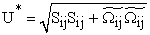(24)

3.2.4. Standard k-ω Turbulence Model

In 1942, Kolmogorov introduced a model with two equations, one based on a transport equation for the turbulent kinetic energy k, the second based on a transport equation for a frequency characteristic of turbulence noted ω. More precisely, the reverse of ω represents the scale of time characteristic of the dissipation rate of the turbulent kinetic energy. The equations for the turbulent kinetic energy k and transport for a frequency characteristic of the turbulence are written as follows:(25)(26)

Where the turbulent viscosity is expressed as a function of k and ω by: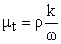(27)
3.3. Combustion Modeling

In this study, we are interested in the turbulent no-premixed flame. Therefore, we have chosen the Eddy Dissipation model to study the reaction rate and also to take account of the turbulence-reaction interaction. This model is based on the Magnussen and Hjertager that have expressed for turbulent diffusion flames when infinitely fast reactions 20. The source term of production of species i due to the reaction is defined by the lesser of the reactant mixing rate and product mixing rate:(28)
3.4. Discretization Procedure

In this paper, we used the commercial CFD code Ansys fluent 17.0 to model the combustion in no-premixed flame methane-air with a simple burner. The numerical solution of the governing equations including mass, momentum, energy, and species was achieved by a finite-volume method. To resolve the governing equations, the simple pressure-velocity coupling algorithm and the upwind discretization scheme were used. Also, we have applied the Eddy dissipation model to take into account the turbulent-reaction interaction. Residuals were reduced to the order of 106 for energy and the others terms of the transport.

3.5. Meshing and Boundary Conditions

In this study, the experimental configuration was studied by Hraiech et al. 11. The aim is to perform a comparison of four turbulence models in a non-premixed turbulent flame with a single burner. The configuration is axis-symmetric. The computational fluid dynamics CFD Ansys fluent 17.0 used a system of cylindrical coordinates. For the mesh, we are interested to compare the numerical results with experimental data. The numerical results with a coarse grid had a large deviation from the experimental results. In fact, with the gradual refining of the grid, the results are stabilized and approached to the experimental values. So, we can confirm that the use of no-uniform grid spacing allows providing a precise resolution close to the axis of the burner where the combustion reaction takes place. In this work, the field is divided into no uniform quadrilateral control volumes for the full domain simulation in order to conserve flux in each cell and to resolve the steep gradients. A total number of 33000 cells with 3434 nodes were used for the simple cylindrical injector. The design of the geometry of the volume control and the mesh generation was performed using the software "GAMBIT" with a quadratic mesh. In the area near the exit of the burner, a refined mesh is considered to fully model the various phenomena that influence in this area. The second order upwind scheme is used to discrete the convective terms of momentum equations, the turbulent kinetic energy and the dissipation rate of the turbulent kinetic energy. The simple algorithm is used for speed correction pressure.

The boundary conditions are determined by the considered experimental configuration as shown in Figure 3. The fuel inlet is related to the output of carburant. So, "velocity inlet" (I) was imposed. The outlet of flame is related to the ambient air that "pressure outlet" (III) was chosen. The inlet and outlet of the oxidant (air) are open to the atmosphere, therefore the conditions "pressure inlet" (II) and "outlet pressure" were imposed respectively on the entry and the outlet. Gas and air temperatures are equal to T=300 K and the atmospheric pressure is equal to p=101325 Pa.

• Figure 3. Geometry mesh

### 4. Experimental Validation

In order to validate the numerical study, the predicted axial and radial velocity profiles are compared to the velocity profiles measurements reported by Hraiech et al. 11. Figure 4 shows the comparison between the numerical results and the experimental data for the considered turbulence models of the axial velocity profiles. The variation of the axial profiles speed on the axis of the jet is divided into two zones. In the first area, potential cone is observed, which disappears at z/d=7.1 of the burner with the k-ε model standard, to z/d = 7.8 with the standard k-ω model and z/d = 7.2 in the experimental measurements. In the second area, the variation of the speed is substantially inversely proportional to the distance z. Also, we see a great match between the numerical results and experimental data. For z>8d, the predicted axial gradients of velocity simulated by the standard k-ε turbulence model present an overall accord when compared to their corresponding models.

• Figure 4. Comparison between numerical results of axial profile and experimental data

The numerical results overestimate the radial velocity profiles compared to the experimental measurement at different positions defined by z = 10 mm, z = 50 mm and z = 100 mm as depicted in Figure 5. In fact, it has been observed that the velocity along the flow decreases and the velocity profile is expanding to the different sections. Near the injector, the profile of the average velocity presents the maximum value equal to V = 18.5 m.s-1 in the downstream, the average speed decreases overall begins. Globally, we find that the results provided by the standard k-ε turbulence model in the three sections are very agreement to the experimental results than the other considered models. In fact, the comparison between the considered turbulence models shows that the standard k-ε turbulence model has better overall results compared to the RNG k-ε and the realizable k-ε models. These results highlight the major differences between the three models which are the method of calculation of the turbulent viscosity, the number of turbulent Prandtl, which orders the turbulent diffusion of k and ε and the terms of generation and destruction in the equation of the dissipation rate of the turbulent kinetic energy. Also, the examination of the results of simulation shows that the standard k-ω turbulence model is not reasonable as that of the standard k-ε turbulence model. Indeed, the standard k-ω turbulence model requires a higher resolution of the grid at the borders, against the standard k-ε is a relatively precise model than we can adopt it for the complex flows.

• Figure 5. Radial profiles of the longitudinal speed at different sections

### 5. Results and Discussion

In this section, the combustion characteristics such as magnitude velocity, static pressure, turbulent kinetic energy, dissipation rate of turbulent kinetic energy and turbulent viscosity was developed under the considered four turbulence models.

5.1. Magnitude Velocity
• Figure 6. Distribution of the axial velocity in r-z plane

The magnitude velocity is calculated for different turbulence models, as depicted in Figure 6. According to these results, it can be seen that there is three interlaced velocity gradient zones in the burner. On the axis of symmetry, the speed is maximal. The jet finds the layer of shearing and the phenomenon of the drive which make it lose its momentum to the profit of the ambient fluid. This loss is reflected in the decline of velocity in the jet at its periphery and by the production of the turbulent kinetic energy. The area bounded by the burner outlet and the position where the axial velocity begins to decrease is called the potential core. The amount of movement introduced by the jet is transferred to the flow in the control volume. The vortex motion in the chamber causes a slight increase in speed near the walls. Globally, we obtain similar results. However, it can be seen that there is a similar distribution at the zone of the potential cone in the standard k-ε, the RNG k-ε, and the standard k-ω models. But, there are a few differences in the velocity distribution inside the combustion chamber and at the walls. Moreover, the reaction is much intensified and well developed with the standard k-ε and the RNG k-ε models.

Figure 7 presents the distribution of the radial velocity component for the different turbulent models. Overall, there are many differences between the distributions of the radial component for the turbulence models using numerical simulation. Outside of the jet, the radial velocity component is equal to zero in the central part. Going downstream, the low-speed values become positive. The maximum value is depicted with the standard k-ε turbulence model which is equal to 0.96 m/s. This fact is dictated by the principle of conservation of mass since the axial velocity decreases in the jet center.

• Figure 7. Radial velocity distribution in the r-z plane
5.2. Static Pressure

The effect of four different turbulence models such as, the standard k-ε, the RNG k-ε, the realizable k-ε and the standard k-ω on static pressure distribution is shown in Figure 8. According to these results, it’s clear that the turbulence model choice has a direct effect on the static pressure distribution. In fact, the static pressure presents tow compression zones characteristics of the maximum value of the static pressure. For the standard k-ε and the RNG k-ε model, the maximal values are obtained just on the exit of the injector and are equal respectively to p= 3.6 Pa and p=2.09 Pa. Moreover, the pressure zone characteristic of the low values is localized in the combustion chamber zone and inside the walls. However, using the realizable k-ε model, the compression zone is extended in the combustion chamber central. In these conditions, the static pressure value is about of p=1060 Pa and the depression zones are localized at the burner inlet.

• Figure 8. Static pressure distribution in the r-z plane
5.3. Turbulent kinetic energy

Figure 9 presents the effect of four different turbulence models on the distribution of the turbulent kinetic energy. From these results, it can be seen that the turbulent kinetic energy is generated on the periphery of the jet shear zone for the standard k-ε, the RNG k-ε and the standard k-ω models. From these results, the turbulent kinetic energy decreases away from the injector and the axis. The thickness of the mixture layer is translated by the width of the peaks of the turbulent kinetic energy. This width increases toward the exit where the energy is dissipated and the flow becomes established. The maximal value is obtained in the central region of the recirculation zone and it’s equal to k=23.5 m2.s-2, k=13.9 m2.s-2 and k=14.1 m2.s-2 respectively for the standard k-ε, the RNG k-ε and the standard k-ω models. Outside of this zone, the turbulent kinetic energy reaches very weak values.

• Figure 9. Turbulent kinetic energy field in different turbulence models
5.4. Dissipation rate of the turbulent kinetic energy

As shown in Figure 10, the dissipation rate of the turbulent kinetic energy distribution is presented for the standard k-ε turbulence model. According to these results, it is clear that the distribution of the dissipation rate of the turbulent kinetic energy is very similar to the turbulent kinetic energy distribution (Figure 8-a). This fact is based on the assumption of the local equilibrium of turbulence, which shows that ε is proportional to k3/2. The standard k-ε model seems most appropriate for determining the characteristics of turbulence. It provides lower levels of turbulent kinetic energy and its dissipation rate compared to other models.

• Figure 10. Distribution of the dissipation rate of the turbulent kinetic energy with the standard k-ε
5.5. Turbulent Viscosity
• Figure 11. Turbulent viscosity distributions in the r-z plane

Turbulent viscosity is an important parameter popularly used to evaluate the combustion characteristics. Figure 11 shows the distribution of the turbulent viscosity for different turbulence models. According to these results, it’s clear that the choice of the turbulence model has a direct effect on the simulation results. In fact, we found that the region characteristic by the low turbulent viscosity is located at the outlet of the injector for the standard k-ε model, the RNG k-ε model and the standard k-ω model. For the standard k-ε model, the high value of the turbulent viscosity appears on the outlet of the combustion chamber and it is equal to= 1.07.10-1 kg.m-1.s-1. For the RNG k-ε model, the characteristic by the high value of the turbulent viscosity is located in the upstream area of the jet with value of about= 9.84 10-2 kg.m-1.s-1. For the standard k-ω the maximal value is obtained inside the combustion chamber and near the lateral walls. The maximum value gotten by this model reaches= 1.16 kg.m-1.s-1. Conversely, the realizable k-ε model gets the minimum at the same area that it’s equal to= 3.72 10-2 kg.m-1.s-1. However, the maximum value is localized at the outlet of the injector and it is equal to= 1.72 kg.m-1.s-1.

### 6. Conclusion

This paper presents numerical results of the turbulence models effect on the combustion characteristics in methane-air diffusion flame. A comparison between four turbulence models including the standard k-ε model, the RNG k-ε model, the realizable k-ε model and the standard k-ω model was performed to get the distribution of the magnitude velocity, the static pressure, the turbulent kinetic energy, the dissipation rate of the turbulent kinetic energy and the turbulent viscosity in a cylindrical burner. The numerical results are analyzed and compared with the experimental results founded from the literature. In these conditions, we confirm that the numerical modeling of the turbulent flow of a diffusion flame, using the standard k-ε model gives more realistic results of a qualitative and quantitative manner. Indeed, confrontation of the axial and the radial profiles of the longitudinal speed with experimental data promotes the use of the standard k-ε turbulence model over other models in our future works.

### References

  J. Fu, Y. Tang, J. Li, Y. Ma, W. Chen and H. Li (2016). Four kinds of the two-equation turbulence model’s research on flow field simulation performance of DPF’s porous media and swirl-type regeneration burner. Applied Thermal Engineering 93, 397-404. In article View Article  A. Ridluan, S. Eiamsa-ard, P. Promvonge(2007). Numerical simulation of 3D turbulent isothermal flow in a vortex combustor. International Communications in Heat and Mass Transfer, 34 860-869. In article View Article  S. Kucukgokoglan, A. Aroussi, S. J. Pickering (1999). Prediction of interaction between burners in multi burner systems. Ph. D. thesis in Nottingham University of Nottingham. In article View Article  I. Yılmaz, M. Tastan, M. Ilbas, C. Tarhan (2013). Effect of turbulence and radiation models on combustion characteristics in propane-hydrogen diffusion flames. Energy Conversion and Management 72, 179-186. In article View Article  A. C. Benim (1990). Finite element analysis of confined turbulent swirling flows. International Journal Numerical Methods Fluids 11, 697-717. In article View Article  H. Yapıcı et al (2005). Numerical calculation of local entropy generation in a methane-air burner. Energy Conversion and Management 46, 1885-1919. In article View Article  L. Ries, J.Carvalho, M.A.R. Nascimento, L.O.Rodrigives, F.Dias ,P.M.Sobrinho (2014). Numerical modeling of flow through an industrial burner orifice. Applied Thermal Engineering 67, 201-213. In article View Article  Z. Riahi, M. A. Mergheni, J.C. Sautet, S. b. Nasrallah. Numerical study of turbulent normal diffusion flame ch4-air stabilized by coaxial burner. Thermal science 17 (2013) 1207-1219. In article View Article  S. J. Brookes, J. B. Moss (1999). Measurements of Soot and Thermal Radiation from Confined Turbulent Jet Diffusion Flames of Methane. Combustion and Flame 116, 49-61. In article View Article  M. Benzitouni, M.S. Boulahlib, Z. Nemouchi (2010). Étude numérique des champs thermique et dynamique des flammes turbulentes premelangées sur un bruleur bunsen. Sciences & technologie 32, 9-16. In article View Article  I. Hraiech, J. C. Sautet, M. A. Mergheni, H. B. Ticha, H.Touati, A. Mhimid (2014). Effects of hydrogen addition and Carbone dioxide dilution on the velocity field in non-reacting and reacting flows. International journal of hydrogen energy, 19818-19831. In article View Article  B.E. Launder and D.B.Spalding (1986). The numerical computation of turbulent flows. Computer methods in applied mechanics and engineering 3, 267-289. In article View Article  B.E. Launder and D.B. Spalding (1972), Lectures in mathematical models of turbulence. Academic Press, London. In article PubMed  D. C. Wilcox (1998). Turbulence Modeling for CFD.DCW Industries. California, Canada. In article  R. Magnussen, B.H. Hjertager (1976). On mathematical modeling of turbulent combustion with special emphasis on soot formation and combustion. In article View Article  S. He, W.S. Kim, J.H. Bae (2008). Assessment of performance of turbulence models in predicting supercritical pressure heat transfer in a vertical Tube. International Journal of Heat and Mass Transfer 51, 4659-4675. In article View Article  T. Deuschle, U. Janoske, M. Piesche, A CFD-model describing filtration, regeneration and deposit rearrangement effects in gas filter systems. Chemical Engineering Journal, 135 (2008) 49-55. In article View Article  T. H. Shih, W.W. Liou, A. Shabbir, Z. Yang, and J. Zhu (1995). A New eddy-viscosity model for high Reynolds number turbulent flows: model development and validation, Computers Fluids 24, 227-238. In article View Article  V. Yakhot and S. A. Orszag (1986). Renormalization Group Analysis of Turbulence: I. Basic Theory, Journal of Scientific Computing, 11-51. In article View Article  Z. Driss, M.S. Abid (2012). Use of the Navier-Stokes Equations to Study of the Flow Generated by Turbines Impellers. Navier-Stokes Equations: Properties, Description and Applications 3, 51-138. In article View Article  Z. Driss, M. Ammar, W. Chtourou, M.S. Abid (2011). CFD Modelling of Stirred Tanks. Engineering Applications of Computational Fluid Dynamics 5, 145-258. In articleThis work is licensed under a Creative Commons Attribution 4.0 International License. To view a copy of this license, visit http://creativecommons.org/licenses/by/4.0/